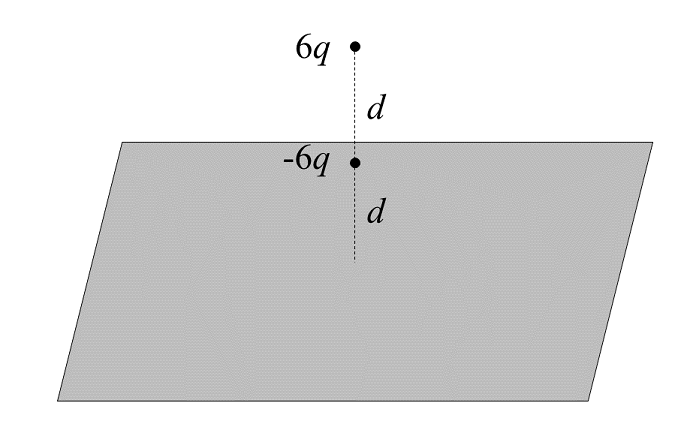# Charges And Conducting SheetTwo charges are placed above an infinite conducting sheet as shown. The first has charge $-6q$ and is placed a distance $d$ above the sheet, whereas the second has charge $6q$ and is placed a distance $d$ directly above the first (so that it is a total distance $2d$ away from the sheet).

Where $k = 1/(4\pi\epsilon_0)$, suppose $F = C \cdot kq^2/d^2$ represents the magnitude of the force on the first charge (of charge $-6q$). What is $C$?

×